# ISEE Middle Level Math : How to find the volume of a net

## Example Questions

### Example Question #1 : How To Find The Volume Of A Net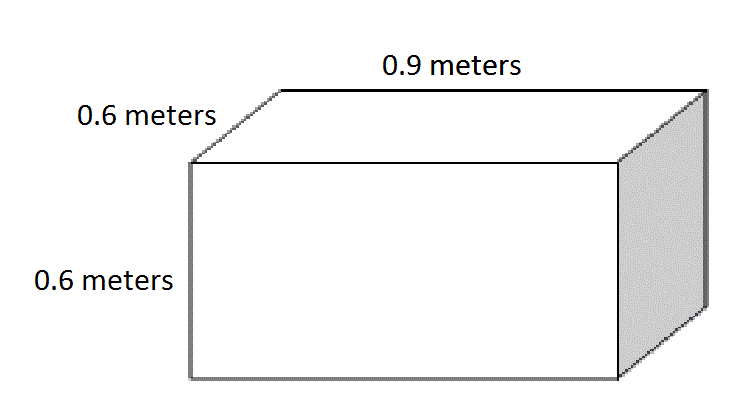Give the volume of the above box in cubic centimeters.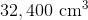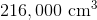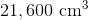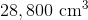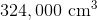Explanation:

100 centimeters make one meter, so convert each of the dimensions of the box by multiplying by 100.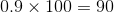centimeters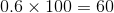centimeters

Use the volume formula, substituting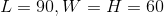: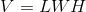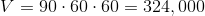cubic centimeters

### Example Question #1 : How To Find The Volume Of A Net

A cube made of nickel has sidelength 20 centimeters, Nickel has a density of 8.9 grams per cubic centimeter. What is the mass of this cube?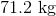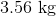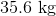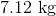Explanation:

The volume of the cube is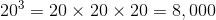cubic centimeters. Multiply by the number of grams per cubic centimeter to get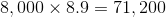grams, or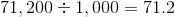kilograms.

### Example Question #1 : Volume Of A Rectangular Solid

A rectangular prism has length 24 inches, width 18 inches, and height 15 inches. Give its volume in cubic feet.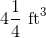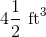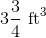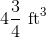Explanation:

Divide each dimension in inches by 12 to convert from inches to feet: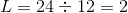feet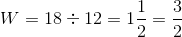feet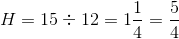feet

Multiply the three to get the volume: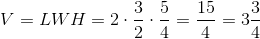cubic feet

### Example Question #3 : Nets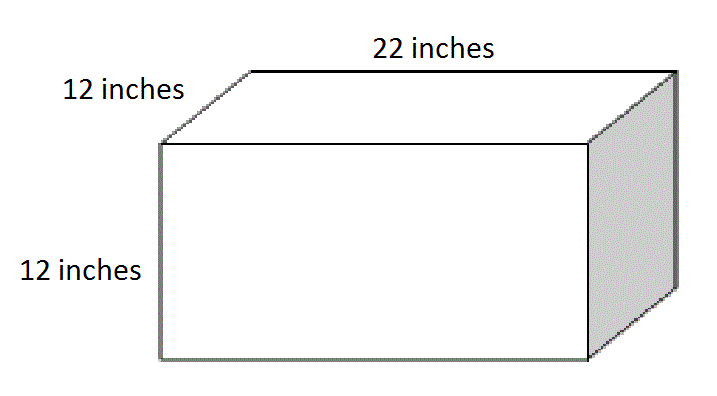Give the volume of the box in cubic inches.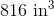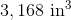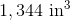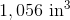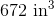Explanation:

Use the volume formula, substituting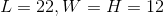: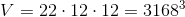### Example Question #4 : Nets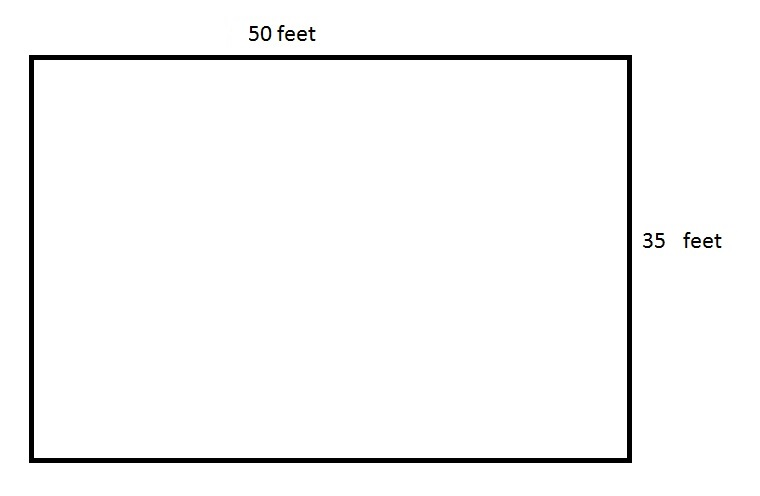One cubic foot is equal to (about) 7.5 gallons.

The above depicts a rectangular swimming pool for an apartment. The pool is seven feet deep everywhere. How many gallons of water does the pool hold?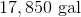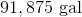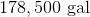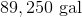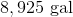Explanation:

The pool can be seen as a rectangular prism with dimensions 50 feet by 35 feet by 7 feet; its volume in cubic feet the product of these dimensions, which is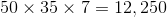cubic feet.

One cubic foot is equal to about 7.5 gallons, so multiply: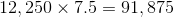gallons.

### Example Question #2 : How To Find The Volume Of A NetThe above depicts a rectangular swimming pool for an apartment. The pool is six feet deep everywhere. What is the volume of the pool in cubic feet?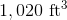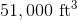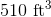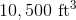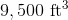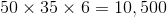cubic feet.